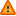FAQ/x1se - CBU statistics WikiYou should trust both wikis because the password could be read by the particular administrators.

Clear message
location: FAQ / x1se

# How do I compute the standard error of X1 in a regression also featuring X2?

There is a closed form expression for this IF X1 and X2 are both centred. That is, have their means subtracted from them.

Centering a variable can reduce collinearity but still give an equivalent fit to the response variable as using the raw data. Centering predictor variables gives the same regression coefficient for the last predictor entered into a regression as using the raw data but a more reliable standard error.

let

A = $$\sum_text{obs i}(X_text{1i}-\mbox{mean})^text{2}$$

B = $$\sum_text{obs i}(X_text{2i}-\mbox{mean})^text{2}$$

C = $$\sum_text{obs i}(X_text{1i}-\mbox{mean})(X_text{2i}-\mbox{mean})$$

D = Mean square residual (amount not explained by X1 and X2)

then

s.e of b(X1) = $$\frac{BD}{AB-C^text{2}}$$

s.e of b(X2) = $$\frac{AD}{AB-C^text{2}}$$

Reference

Aiken and West (1991) Multiple Regression: Testing and Interpreting Interactions.